Published: 31 March 2014

# A new model for artificial seismic wave synthesis

Xu Qiang1
Chen Jian-yun2
Li Jing3
Xu Gang4
1, 2, 3School of Civil and Hydraulic Eng., Dalian University of Technology, Dalian, China
Corresponding Author:
Xu Qiang
Views 118

#### Abstract

A new model is proposed based on wavelet theory and genetic algorithms (GAs) in order to improve precision of artificial seismic wave. This model was mainly divided into three parts. Firstly, Mallat method was used to decompose power spectral density function with wavelet base. Then the initial artificial seismic wave was synthesized based on wavelet theory. Thirdly, the iteration processes of artificial seismic wave synthesis were optimized by genetic algorithms. Two numerical examples were given. The first numerical example mainly focuses on the analysis for the initial artificial seismic wave synthesis based on wavelet theory. And the second example mainly focuses on the analysis for the iterative process of artificial seismic wave synthesis based on genetic algorithms. Compared with the conventional method of cosine superposition, this model has smaller error between the calculated acceleration response spectrum and the target response spectrum and can be applied in engineering.

## 1. Introduction

The theory and methods for artificial seismic wave synthesis have been developed significantly during the last thirty years and have been documented in an increasing number of publications. In order to simulate artificial seismic wave, a rigorous series of tests has to be carried out.

Using the transition relationship between the response spectrum and the power spectrum, Chen et al. (1983)  synthesized artificial earthquake ground motions fitted with the standard response spectrum. Considering phase spectrum through the phase correction technique, Hu and He (1986)  suggested a simulated ground motion method from the response spectrum. Shinozuka and Deodatis  (1991) developed an analytical model for propagating seismic waves that could account for their stochastic characteristics in the time and space domain. From this model, the corresponding sample seismic waves could be digitally generated with great computational efficiency. Conte et al.  (1992) used discrete time-varying auto regressive moving average (ARMA) models to describe realistic earthquake ground motion time histories. Deodatis  (1996) presented a spectral-representation-based simulation algorithm to generate sample functions of a non-stationary stochastic process with evolutionary power, according to its prescribed non-stationary cross-spectral density matrix. Numerous studies, including those made by Anderson  (1987), Hall  (1995), and Macrae  (2001), indicated that structures experience increased inelastic demands when subjected to near-field ground motions. Zhang and Lou  (2001) established a generalized earthquake-wave-motion model, which considered 3D random heterogeneous media, together with existing models for source and realistic geological profiles for sedimentary basins and irregular topography. Both amplitude and frequency non-stationary were incorporated in the model. Zerva and Zervas  (2002) suggested that an appropriate simulation technique could be successful in matching the characteristics of the simulated motions with those of the target field. Based on the phase difference spectrum, generating a non-stationary ground motion in both the time and frequency domains was proposed by Yang and Jiang (2002) . Dong et al.  (2007) presented an improved local convergent method to simulate multi-point earthquake ground motions. Manoj and Singh  (2009) presented a modal to evaluate and predict the blast-induced ground vibration and frequency by incorporating rock properties, blast design and explosive parameters using the artificial neural network (ANN) technique.

Wavelet analysis was applied to engineering problems by several authors. Newland [13, 14] (1994) used wavelets to analyze structural vibrations due to underground and surface traffic. Gurley and Kareem  (1999) examined a variety of applications for wavelet analysis including two general methods for simulating non-stationary processes. If a target power spectrum was given, a different modulating function could be used for each wavelet frequency band following a procedure similar to Priestley’s evolutionary spectrum  (1965). The procedure proposed by Iyama  (1999) was similar to the latter method. A bilinear target spectrum was used for simplicity and the modulating functions, which were expressed as energy release rates. Legrue and Menun  (2004) described a procedure that utilized the wavelet decomposition of a recorded acceleration to simulate an ensemble of ground motions that had localized temporal features and variations in the frequency content that were similar to those present in the original record.

In this paper, wavelet theory and genetic algorithms (GAs) are used for establishing the new model for artificial seismic wave synthesis in order to decrease the error between the target response spectrum and the calculated response spectrum of artificial seismic wave.

## 2. The establishment for artificial seismic wave synthetic model

### 2.1. The initial artificial seismic wave synthesis based on wavelet theory

According to wavelets multi-resolution analysis theory, $\left\{{V}_{n};n\in Z\right\}$ is the orthogonal multi-resolution analysis of scaling function $\phi$, the scaling relation (1) is given as:

1
$\phi \left(\omega \right)=\sum _{k\in Z}{h}_{k}\phi \left(2\omega -k\right),$

in which:

2
${h}_{k}=2{\int }_{-\infty }^{\infty }\phi \left(\omega \right)\stackrel{-}{\phi \left(2\omega -k\right)}dx,$

and

3
$\phi \left({2}^{j-1}\omega -l\right)=\sum _{k\in z}{h}_{k-2j}\phi \left({2}^{j}\omega -k\right).$

It is assumed that ($\left\{{V}_{m};m\in Z\right\}$; $\phi \left(\omega \right)$) is the orthogonal multi-resolution analysis, then $\left\{{h}_{k}\right\}\in {l}^{2}$ can be found to make the Eq. (4) exist:

4
$\phi \left(\omega \right)=\sum _{k}{h}_{k}\phi \left(2\omega -k\right).$

The scaling function $\phi \left(\omega \right)$ is used to construct function:

5
$\psi \left(\omega \right)=\sum _{k}{g}_{k}\phi \left(2\omega -k\right).$

The dilation and translation of $\psi \left(\omega \right)$ constructs orthogonal basis of ${L}^{2}\left(R\right)$, in which:

6
${g}_{k}={\left(-1\right)}^{k}{\stackrel{-}{h}}_{1-k},$

and

7
${W}_{j}\perp {W}_{{j}^{\text{'}}},j\ne {j}^{\text{'}},{W}_{j}\oplus {V}_{j}={V}_{j+1}$

when:

${W}_{j}=span\left\{{2}^{j/2}\psi \left({2}^{j}x-k\right);k\in Z\right\}$

where $\psi \left(\omega \right)$ is defined as wavelet function.

Mallat method is used to decompose power spectral density function ${S}_{x}\left(\omega \right)$ with wavelet. The basic idea is ${H}_{j}{S}_{x}\left(\omega \right)$, which is defined as the approximation under resolving capability ${2}^{j}$ of finite energy signals, can be further decomposed into ${H}_{j-1}{S}_{x}\left(\omega \right)$ and ${D}_{j-1}{S}_{x}\left(\omega \right)$, which is defined as the detail between resolving capability ${2}^{j}$ and ${2}^{j-1}$. The decomposition course is shown as Fig. 1.

Fig. 1The decomposition process of signals at different frequency band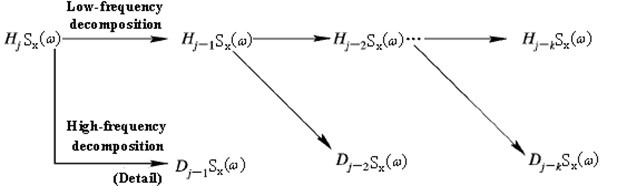The coefficient of two-scale equations $\left\{{h}_{k}\right\}$, $\left\{{g}_{k}\right\}$ can be obtained as:

8
${h}_{m-2n}=\sqrt{2}〈{\phi }_{j-1,n}{\phi }_{j,m}〉,$
9
${g}_{m-2n}=\sqrt{2}〈{\psi }_{j-1,n}{\phi }_{j,m}〉.$

And Eq. (10), (11) and (12) can be obtained as:

10
${H}_{j-1}{S}_{x}\left(\omega \right)=\sum _{k=-\infty }^{+\infty }{a}_{k}^{j-1}\phi \left({2}^{j-1}\omega -k\right),$
11
${D}_{j-1}{S}_{x}\left(\omega \right)=\sum _{k=-\infty }^{+\infty }{d}_{k}^{j-1}\psi \left({2}^{j-1}\omega -k\right),$
12
${H}_{j}{S}_{x}\left(\omega \right)=\sum _{k=-\infty }^{+\infty }{a}_{k}^{j}{\phi }_{j,k}\left(\omega \right).$

Then the stationary process ${a}_{s}\left(t\right)$ by wavelet basis is given as:

13
${a}_{s}\left(t\right)=\sum _{k=1}^{N}\left(\begin{array}{c}\sqrt{4{H}_{j-r}{S}_{x}\left({\omega }_{k}\right)\mathrm{\Delta }\omega }cos\left({\omega }_{k}t+{\varphi }_{k,l}\right)\\ +\sum _{i=1}^{{r}_{1}}\left(\sqrt{4{D}_{j-i}{S}_{x}\left({\omega }_{k}\right)\mathrm{\Delta }\omega }\mathrm{c}\mathrm{o}\mathrm{s}\left({\omega }_{k}t+{\varphi }_{k,l}\right)\right)\\ +\sum _{l={r}_{1}}^{r}\left(\sqrt{4{D}_{{r}_{1}-l}{S}_{x}\left({\omega }_{k}\right)\mathrm{\Delta }\omega }\mathrm{c}\mathrm{o}\mathrm{s}\left({\omega }_{k}t+{\varphi }_{k,l}\right)\right)\end{array}\right),$

where ${\mathrm{\Phi }}_{k,l}$ is the random phase angle obeyed homogeneous distribution.

And the power spectral density function ${S}_{x}\left(\omega \right)$ is given as:

14
$\left\{\begin{array}{l}{S}_{x}\left({\omega }_{k}\right)=\frac{2\xi }{\pi {\omega }_{k}}{\left[{S}_{a}^{T}\left({\omega }_{k}\right)\right]}^{2}\frac{1}{-2\mathrm{ln}\left(-\frac{\pi }{{\omega }_{k}{T}_{d}}\mathrm{l}\mathrm{n}p\right)},\\ \mathrm{\Delta }\omega =2\pi /{T}_{d},\\ {\omega }_{k}=\mathrm{\Delta }\omega k,k=1,2,3,\dots ,n,\\ n=\frac{{T}_{d}}{∆t},\end{array}\right\$

where ${S}_{a}^{T}\left({\omega }_{k}\right)$ is the target response spectrum, $p$ is the probability of the calculated acceleration response spectrum greater than the target response spectrum, $\xi$ is damping ratio, $∆t$ is time step. The ${S}_{a}^{T}\left({\omega }_{k}\right)$ is shown as Fig. 2. In Fig. 2, ${T}_{g}$ is the characteristic period of ground and $\beta$ is amplification factor.

Fig. 2The target response spectrum SaT(ωk)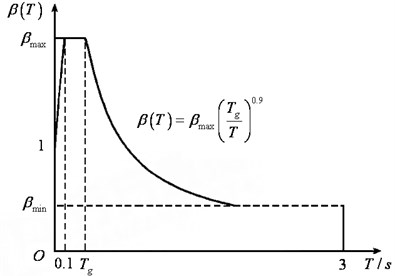In order to reflect the non-stationary seismic wave ${\stackrel{¨}{x}}_{g}\left(t\right)$, ${a}_{s}\left(t\right)$ multiplied by the envelope function $f\left(t\right)$ is adopt as:

15
${\stackrel{¨}{x}}_{g}\left(t\right)=f\left(t\right)a\left(t\right),$

where $f\left(t\right)$ is given as:

16
$f\left(t\right)=\left\{\begin{array}{ll}\frac{{t}^{2}}{{t}_{1}^{2}},& 0\le t<{t}_{1},\\ 1,& {t}_{1}\le t<{t}_{2},\\ \mathrm{e}\mathrm{x}\mathrm{p}\left(-{c}_{d}\left(t-{t}_{2}\right)\right),& {t}_{2}\le t<{t}_{3},\end{array}\right\$

where the range of attenuation coefficient ${c}_{d}$ is 0.1-1.0. ${t}_{1}$ and ${t}_{2}$ are the first and last time of the stationary process, respectively. ${t}_{3}$ is the duration of seismic wave, usually ${T}_{d}={t}_{3}$. In order to improve the frequency resolution, it usually makes ${T}_{d}>{t}_{3}$ and $f\left(t\right)=0$ when ${t}_{3}\le t\le {T}_{d}$.

### 2.2. The iterative process of artificial seismic wave synthesis based on genetic algorithms

Genetic algorithms (GAs) are adaptive heuristic search algorithm premised on the evolutionary ideas of natural selection and genetic. Algorithm is started with a set of solutions (represented by chromosomes) called population. Solutions from one population are taken and used to form a new population. This is motivated by a hope, that the new population will be better than the old one. Solutions which are selected to form new solutions (offspring) are selected according to their fitness-the more suitable they are the more chances they have to reproduce. This is repeated until some condition (for example number of populations or improvement of the best solution) is satisfied. Genetic algorithms are usually used for solving the optimization problem, whose objective function is implicit expression. The details on seismic wave synthesis based on genetic algorithms are discussed as follow.

Because approximate transformational relation between the target response spectrum and the power spectrum is adopted in the initial step of artificial seismic wave synthesis, the numerical iterative process of calculated acceleration response spectrum ${S}_{a}\left({\omega }_{k}\right)$ must be supplemented in order to fit the target response spectrum ${S}_{a}^{T}\left({\omega }_{k}\right)$.

The error $E\left({\omega }_{k}\right)$ between ${S}_{a}^{T}\left({\omega }_{k}\right)$ and ${S}_{a}\left({\omega }_{k}\right)$ at frequency ${\omega }_{k}$ is defined as:

17
$E\left({\omega }_{k}\right)={‖{S}_{a}\left({\omega }_{k}\right)-{S}_{a}^{T}\left({\omega }_{k}\right)‖}_{2}.$

The total error $ET$ is defined as:

18
$ET=\frac{1}{N}\sum _{k=1}^{N}E\left({\omega }_{k}\right),$

where $N$ is the number of ${\omega }_{k}$.

The iteration step $i$ of ${S}_{x}\left({\omega }_{k}\right)$ is calculated as:

19
${\left(\sqrt{{S}_{x}\left({\omega }_{k}\right)}\right)}_{i+1}={\alpha }_{k}{\left(\sqrt{{S}_{x}\left({\omega }_{k}\right)}\right)}_{i}\frac{{S}_{a}^{T}\left({\omega }_{k}\right)}{{S}_{a}\left({\omega }_{k}\right)},k=1,2,\cdots ,N,$

where ${\alpha }_{k}$ are modifying weights at frequency ${\omega }_{k}$.

When ${\alpha }_{k}$ and $ET$ are respectively used as the optimization variables and minimal optimization goals, ${\alpha }_{k}$ are given through solving the following optimization problem as:

20
$\left\{\begin{array}{l}\text{Find}{\alpha }_{k},\\ \mathrm{m}\mathrm{i}\mathrm{n}E{T}_{i+1}=\frac{1}{N}\sum _{k=1}^{N}{E}_{i+1}\left({\omega }_{k}\right)=\frac{1}{N}\sum _{k=1}^{N}{‖{S}_{a}{\left({\omega }_{k}\right)}_{i+1}-{S}_{a}^{T}\left({\omega }_{k}\right)‖}_{2},\\ {|{C}_{k}|}_{i+1}={\alpha }_{k}{|{C}_{k}|}_{i}\frac{{S}_{a}^{T}\left({\omega }_{k}\right)}{{S}_{a}{\left({\omega }_{k}\right)}_{i}}.\end{array}\right\$

If the maximum value of $E\left({\omega }_{k}\right)$ need to control, the optimization problem (20) can be revised as:

21
$\left\{\begin{array}{l}\text{Find}\text{}{\alpha }_{k},\\ \mathrm{m}\mathrm{i}\mathrm{n}E{T}_{i+1}=\frac{1}{N}\sum _{k=1}^{N}{E}_{i+1}\left({\omega }_{k}\right)+Qmax{E}_{i+1}\left({\omega }_{k}\right),\\ {|{C}_{k}|}_{i+1}={\alpha }_{k}{|{C}_{k}|}_{i}\frac{{S}_{a}^{T}\left({\omega }_{k}\right)}{{S}_{a}{\left({\omega }_{k}\right)}_{i}},\end{array}\right\$

where $Q$ is penalty function.

The objective functions of optimization problem (20) or (21) are implicit expressions, so GAs can be used to solve them.

Through solving optimization problem (20) or (21) by GAs, ${\alpha }_{k}$ can be obtained. Through substituting ${\alpha }_{k}$ into Eq. (19) and then substituting Eq. (19) into Eq. (13) and (15), the iterative process of artificial seismic wave synthesis can be calculated.

## 3. Numerical analysis

### 3.1. Numerical example 1

This section mainly focuses on the analysis for the initial artificial seismic wave synthesis based on wavelet theory. The parameters of the initial artificial seismic wave are given as follow: The first and last time of the stationary process are given as ${t}_{1}=$2 s, ${t}_{2}=$16 s. The duration of seismic wave is given as ${t}_{3}=$20 s. The attenuation coefficient is given as ${c}_{d}=$0.80. The maximum value of amplification factor is given as ${\beta }_{max}=$2.0. The characteristic period of ground is given as ${T}_{g}=$0.40. The time step is given as $∆t=$0.01 s. The probability of the calculated acceleration response spectrum greater than the target response spectrum is given as $p=$0.15. The damping ratio is given as $\xi =$0.05. The peak acceleration is 0.251$g$ where $g$ is the acceleration of gravity. The initial artificial seismic wave is synthesized as Daubechies wavelet base (db1) and Daubechies wavelet base (db4), and the power spectral density function ${S}_{x}\left(\omega \right)$ is decomposed to 4 levels.

The initial artificial seismic wave generated by conventional method of cosine superposition and the calculated response spectrum of which are shown as Fig. 3 and 4, respectively. The initial artificial seismic wave generated by wavelet base (db1) method and the calculated response spectrum of which are shown as Fig. 5 and 6, respectively. The initial artificial seismic wave generated by wavelet base (db4) method and the calculated response spectrum of which are shown as Fig. 7 and 8, respectively.

Fig. 3The initial artificial seismic wave generated by conventional method of cosine superposition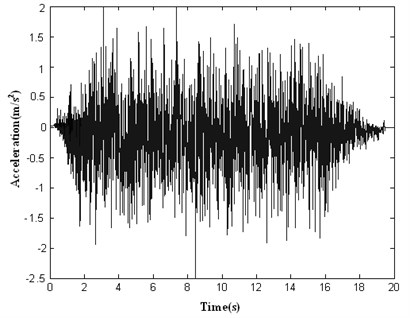Fig. 4Response spectrum of initial artificial seismic wave generated by conventional method of cosine superposition compared with the target response spectrum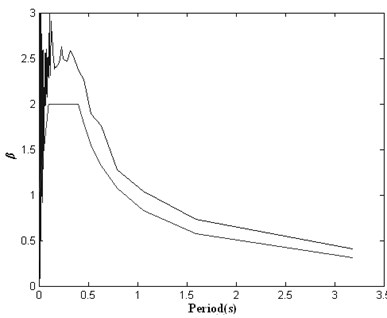### 3.2. Numerical example 2

This section mainly focuses on the analysis for the iterative process of artificial seismic wave synthesis based on genetic algorithms. The parameters of the initial artificial seismic wave are given as follow: The first and last time of the stationary process are given as ${t}_{1}=$2 s, ${t}_{2}=$8 s. The duration of seismic wave is given as ${t}_{3}=$16 s. The attenuation coefficient is given as ${c}_{d}=$0.80. The maximum value of amplification factor is given as ${\beta }_{max}=$2.0. The characteristic period of ground is given as ${T}_{g}=$0.40. The time step is given as $∆t=$0.01 s. The probability of the calculated acceleration response spectrum greater than the target response spectrum is given as $p=$0.15. The damping ratio is given as $\xi =$0.05. The peak acceleration is 0.251$g$ where $g$ is the acceleration of gravity.

Fig. 5The initial artificial seismic wave generated by wavelet base (db1) method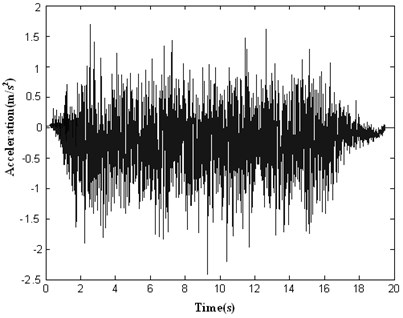Fig. 6Response spectrum of initial artificial seismic wave generated by wavelet base (db1) method compared with the target response spectrum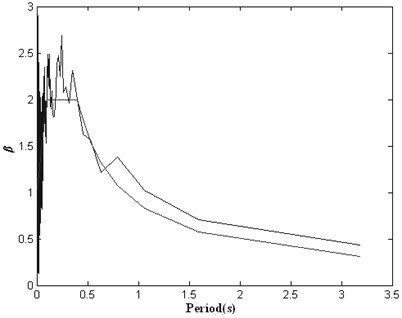Fig. 7The initial artificial seismic wave generated by wavelet base (db4) method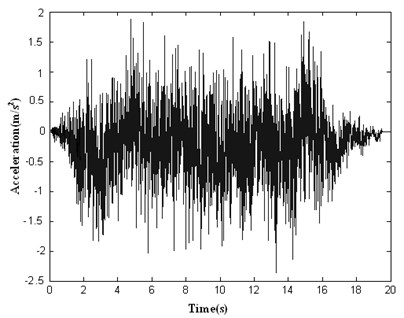Fig. 8Response spectrum of initial artificial seismic wave generated by wavelet base (db4) method compared with the target response spectrum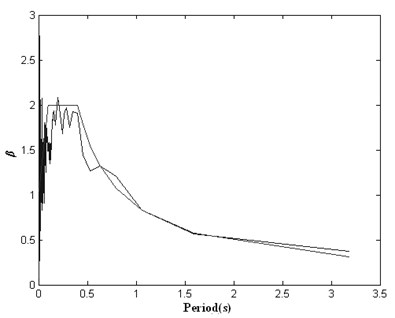Fig. 9The artificial seismic waves generated by the conventional method of cosine superposition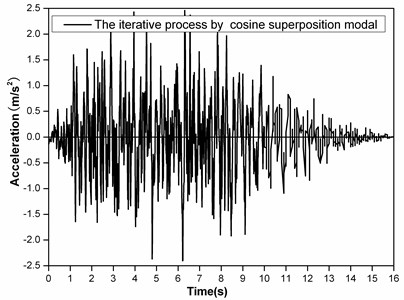Fig. 10The artificial seismic waves generated by this paper’s method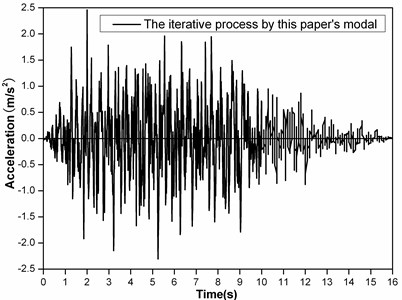The artificial seismic waves generated by the conventional method of cosine superposition and this paper’s method based on GAs are shown as Fig. 9 and 10, respectively. The calculated response spectrums of artificial seismic waves generated by the conventional method of cosine superposition and this paper’s method compared with the target response spectrum are shown as Fig. 11. The total error with iteration steps are shown as Fig. 12.

Fig. 11The response spectrums of artificial seismic waves generated by the conventional method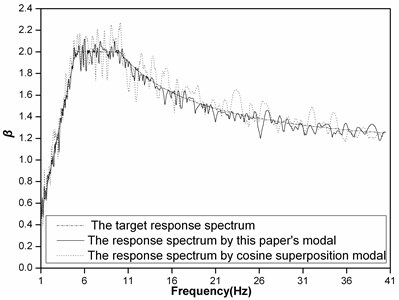Fig. 12The total error with iteration steps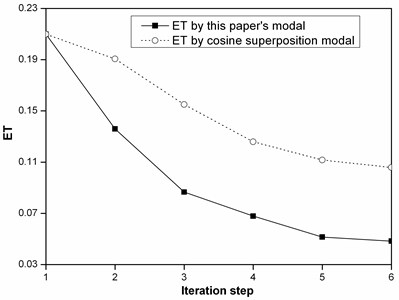## 4. Discussion

From the analysis of Fig. 3-8, it shows that Daubechies wavelet base (db4) is better than Daubechies wavelet base (db1) to generate initial artificial seismic wave comparing with the effect of using these wavelet bases. And the total error by using Daubechies wavelet base is smaller than by using the conventional method of cosine superposition. From the analysis of Fig. 9-12, it shows that the total error by using by using this paper’s model based on GAs is 3 times smaller than the conventional method of cosine superposition. And the descent speed of the total error by using this paper’s model based on GAs is higher than by using the conventional method of cosine superposition. From what has been discussed above, it shows that this paper’s model can improve precision of artificial seismic wave and can be applied in engineering.

## 5. Conclusion

Artificial seismic wave synthesis model is established based on wavelet theory and genetic algorithm. The initial artificial seismic wave synthesis is optimized by wavelet theory. The iteration processes of artificial seismic wave synthesis are optimized by genetic algorithms. The result showed that the total error between calculated and target response spectrum by this paper’s method is smaller than by conventional method of cosine superposition.